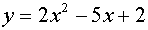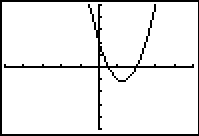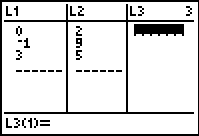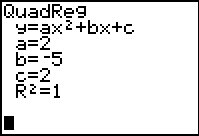Find the solutions (roots) of a quadratic equation using the graphing calculator.
Consider the following examples:

 1. Using the ZERO Command Solve: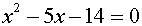Since this equation is set equal to zero, the roots will be the locations where the graph crosses the x-axis (if the roots are real numbers). (Remember that the x-axis is really just  y = 0.) 1.  Set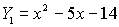2.  Use the ZERO command to find the roots --  2nd TRACE (CALC), #2 zero 3.  Left bound?  Move the spider as close to the root (where the graph crosses the x-axis) as possible.  Hit the left arrow to move to the "left" of the root.  Hit ENTER.  A "marker" ► will be set to the left of the root. 4.  Right bound?  Move the spider as close to the root (where the graph crosses the x-axis) as possible.  Hit the right arrow to move to the "right" of the root.  Hit ENTER.  A "marker" ◄ will be set to the right of the root. 5.  Guess?  Just hit ENTER. 6.  Repeat the entire process to find the second root (which in this case happens to be x = 7). Answer:  one of the roots is x = -2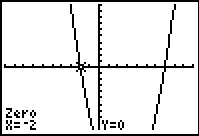Standard 10 x 10 window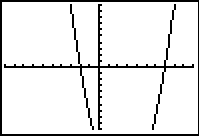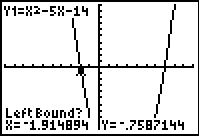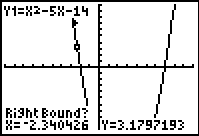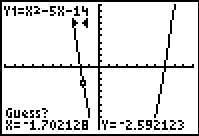2. Using the INTERSECT Command Solve: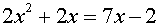Since this equation is NOT set equal to zero, the ZERO command cannot be used to look for roots(unless you re-write the equation so that it IS set equal to zero). If you do not want to re-write the equation, solve using the intersect command to find the points where the two expressions intersect (if the roots are real numbers): 1.  Set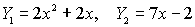2.  Use the intersect command to find the roots, 2nd TRACE (CALC), #5 intersect. 3.  First Curve?  Move the spider close the point of intersection that you want to find.  Hit ENTER. 4.  Second Curve? Just hit ENTER.  5.  Guess?  Just hit ENTER. 6.  Repeat the entire process to find the second root (which in this case happens to be x = 2). Answer:  one of the roots is x = 0.5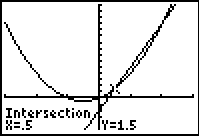Window [-3,3] x [-5,15]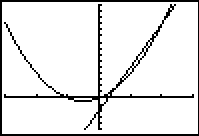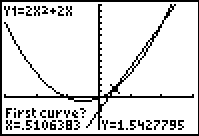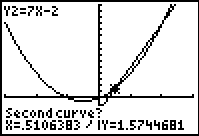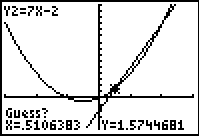3.
Only One Root?

Solve: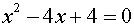When graphed, this equation only intersects the x-axis in one location.  This tells you that this root repeats itself.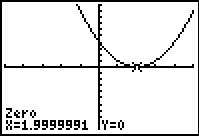If a reading appears such as 1.9999991, the actual answer is most likely the number 2.  Check your assumption that the answer is 2 by substituting 2 into the original equation to see if it satisfies the equation.
Standard 10 x 10 window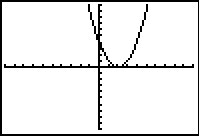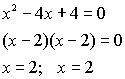4. What if the graph does not intersect the x-axis??? (or intersect option shows no intersections) Solve: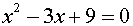When graphed, this equation does NOT intersect the x-axis.  This tells you that the roots of this equation are complex (imaginary) values.  Dig out the quadratic formula to find the roots.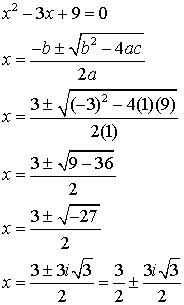Window [-5,5] x [-5,15]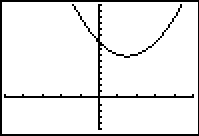5. Need to WRITE a quadratic equation from a graph? If you are given the visual graph of a quadratic equation and you are given (or can identify) at least 3 points, you can use the Quadratic Regression process to create the equation of the graph. Write the equation of the parabola shown at the right, given that the points (0,2), (-1,9) and (3,5) are on the graph. 1.  Enter the points into L1 and L2 (x-coordinate in L1 and matching y-coordinate in L2) (See Basic Commands for Stats if you need more assistance.) 2.  Press STAT.  Arrow over to CALC.  Choose #5 QuadReg. 3.  On the home screen:  QuadReg L1, L2 4.  Press ENTER.  You will see the screen at the right showing you the coefficients and constant of the quadratic equation. This equation is